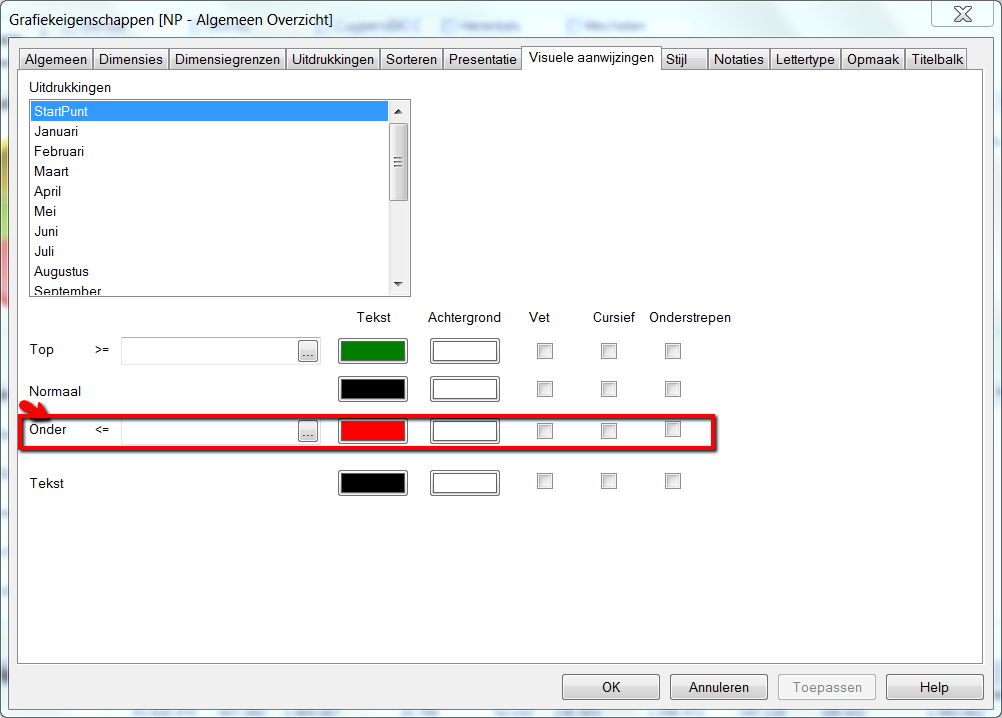# New to QlikView

Discussion board where members can get started with QlikView.

Announcements

Contributor II

## Need easy way for calculations

Hi all,

I'm New to Qlikview and i need to have below calculation,

 quntity difference Jan 15 feb 20 5 march 18 -2 april 40 22

Like Thant.

I want to get the different with out adding a new feild in load statement?

Is there a way to get it?

Thanks

1 Solution

Accepted SolutionsMVP

## Re: Need easy way for calculations

Try using chart inter record functions, like above().

I assume you've created a chart with a month dimension, and an expression with something like sum(Quantity).

The diff column could then look like

=sum(Quantity) - above(sum(Quantity))

8 RepliesMVP

## Re: Need easy way for calculations

Try using chart inter record functions, like above().

I assume you've created a chart with a month dimension, and an expression with something like sum(Quantity).

The diff column could then look like

=sum(Quantity) - above(sum(Quantity))

Contributor

## Re: Need easy way for calculations

Create a table

with as dimension the months

and as expresions:

- Quantity with formula sum(if(month = '02',products,0))

- Difference with formula sum(if(month = '02',products,0)) - sum(if(month = '01',products,0))

This solved my problem.

Regards,

A.

Contributor II

## Re: Need easy way for calculations

Thanks, This works nice for me. Another one thing.

If i need to show - values in red color how can i do it?

Thanks For your helpMVP

## Re: Need easy way for calculations

Hi

In expression tab, click the '+' sign and give text color or background color for your condition using if() or give simply RGB(124,123,123)

Hope it helps

Contributor II

## Re: Need easy way for calculations

i need to have Red colour for this,

so how to use if condition with that.

if (sum(Quantity) - above(sum(Quantity)) < 0) then how to get the red colorMVP

## Re: Need easy way for calculations

Hi

Try like this,

Expression tab -> click '+' sign -> text color or background color -> in expression

=

if (sum(Quantity) - above(sum(Quantity)) < 0, Red())

Hope it helps

Contributor II

Great, Thanks

Contributor

## Re: Need easy way for calculations

Hi,

Why don't you use, the tabpage

presentation or visual styles.

See the screenshot belowCommunity Browser